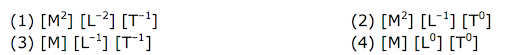# If E and G respectively denote energy and gravitational constant, then E /G has the dimensions of:

If E and G respectively denote energy and gravitational constant, then $$E\over G$$ has the dimensions of:verified

Correct answer: (2) [M2] [L-1] [T0]

Explanation:

E = energy = [ML2T-2]

G = Gravitational constant = [M-1L3T-2]

So $$\frac{E}{G} = \frac{[E]}{[G]}$$

$$ML^2T^{−2}\over M^{−1}L^3T^{−2}$$ = [M2] [L-1] [T0]